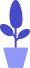# Python turtle module example: analysis before drawing peppa pig

Estimated reading: 4 minutes 68 views

In the previous tutorial, we drew a cartoon image of a robot cat with a turtle drawing. Now let’s introduce how to use turtle drawing to draw another cartoon character that children like – Peppa Pig.

## program analysis

Let’s first take a look at what Peppa Pig looks like, as shown in Figure 1.

Looking at this image, you can see that Peppa Pig is basically composed of various curves. Her nose is an oval. The head is made up of several arcs connected, and the ears are also made up of several arcs. The eyes are big circles over small circles. The gill is a solid circle. The mouth is an arc. Then there is the body, with two curved lines on the left and right, and a straight line below. Arms can be represented by straight lines and fingers by curved lines. We can also use two vertical lines to represent the left and right legs, and two horizontal lines to represent the feet. Finally use 3 arcs to draw the little tail with curls.

We still use custom functions to define each drawing function according to the parts of Peppa Pig: nose (nose), head (head), ears (ears), eyes (eyes), cheek (gills), mouth (mouth), body, hands, foot and tail. The name of the function indicates which part of the body the function is responsible for drawing.

## Draw arcs and ellipses

It can be seen that many parts are drawn with arcs. As we mentioned when we introduced the circle() function before, this function has three parameters, namely radius (radius), extent (radian) and steps (making the inscribed regular polygon of a circle with radius radius), of which the latter Both parameters are optional.

Previously we used the circle() function to draw circles, so either pass only one parameter to this function, or set it to 360 when passing the second parameter. If you use the circle() function to draw an arc, specify the corresponding radian for the second parameter.

Let’s see how to draw an arc through a simple example, the code is as follows.

``````import turtle
turtle.pencolor("red")
turtle.circle(100,120)``````

The execution result is shown below, and a red arc is drawn in the window, as shown in Figure 2.figure 2
Because there is no function to directly draw an ellipse in turtle, we need to change the way of thinking. If we want to use a regular polygon to simulate a circle, when there are enough sides, a very close circle can be simulated. For example, a regular 120-sided shape looks very close to a circle. Let’s try to do it, the code is as follows.

``````import turtle
turtle.pendown()
for j in range(120): # Repeat 120 times
turtle.forward(5) # move 5 pixels
turtle.left(3) # Turn left 3 degrees
turtle.penup()``````

We execute a loop 120 times, moving 5 pixels in each loop, then turning left 3 degrees. After this completes the loop, it turns exactly 360 degrees to the left and returns to the original position. During the execution, in the window, we will see that the cursor starts from the initial position and draws an approximate circle counterclockwise, as shown in Figure 3.image 3
If you modify the parameters in forward(), you can also draw circles with different radii. This gives us more freedom. In the range of angles, the speed of arc drawing is affected by modifying the parameters in forward().

We still draw 120 arcs, but this time through the if-else conditional statement, the first 30 steps make the arc speed from slow to fast, the next 30 steps from fast to slow, and the next 30 steps let the arc draw The speed is again from slow to fast, and the speed of the last 30 steps is from fast to slow, so that the uneven painting method can form an ellipse.

Let’s try to do it, the code is as follows.

``````import turtle
turtle.pendown()
segment=1
for i in range(120):
if 0<=i<30 or 60<=i<90:
segment= segment+0.2
turtle.left(3)
turtle.forward(segment)
else:
segment= segment-0.2
turtle.left(3)
turtle.forward(segment)``````

Running this code, you can see that the turtle draws an ellipse, as shown in Figure 4.Figure 4

## import turtle module

From here, we’ll introduce how to draw Peppa Pig, and in the same case as mentioned before, to use turtle drawing, we need to import the turtle module first. We still use the second method of importing modules introduced earlier:

``````from turtle import *
``````

In this way, all the methods and variables in the turtle module can be imported, and then the methods can be called directly without adding the “turtle.” prefix.